# High Dimensional Data Visualization

## Serialaxes coordinate

Serial axes coordinate is a methodology for visualizing the $$p$$-dimensional geometry and multivariate data. As the name suggested, all axes are shown in serial. The axes can be a finite $$p$$ space or transformed to an infinite space (e.g. Fourier transformation).

In the finite $$p$$ space, all axes can be displayed in parallel which is known as the parallel coordinate; also, all axes can be displayed under a polar coordinate that is often known as the radial coordinate or radar plot. In the infinite space, a mathematical transformation is often applied. More details will be explained in the sub-section Infinite axes

A point in Euclidean $$p$$-space $$R^p$$ is represented as a polyline in serial axes coordinate, it is found that a point <–> line duality is induced in the Euclidean plane $$R^2$$ (Inselberg and Dimsdale 1990).

Before we start, a couple of things should be noticed:

• In the serial axes coordinate system, no x or y (even group) are required; but other aesthetics, such as colour, fill, size, etc, are accommodated.

• Layer geom_path is used to draw the serial lines; layer geom_histogram, geom_quantiles, and geom_density are used to draw the histograms, quantiles (not quantile regression) and densities. Users can also customize their own layer (i.e. geom_boxplot, geom_violin, etc) by editing function add_serialaxes_layers.

### Finite axes

Suppose we are interested in the data set iris. A parallel coordinate chart can be created as followings:

library(ggmulti)
# parallel axes plot
ggplot(iris,
mapping = aes(
Sepal.Length = Sepal.Length,
Sepal.Width = Sepal.Width,
Petal.Length = Petal.Length,
Petal.Width = Petal.Width,
colour = factor(Species))) +
geom_path(alpha = 0.2)  +
coord_serialaxes() -> p
p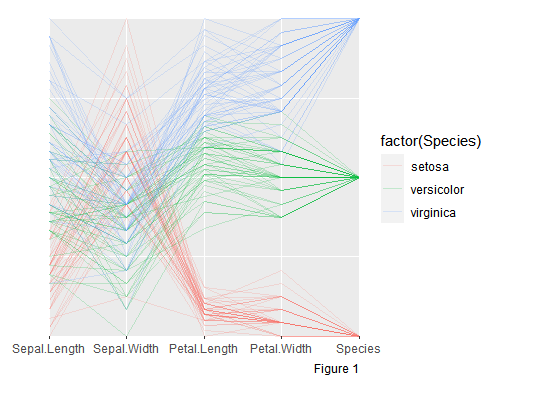A histogram layer can be displayed by adding layer geom_histogram

p +
geom_histogram(alpha = 0.3,
mapping = aes(fill = factor(Species))) +
theme(axis.text.x = element_text(angle = 30, hjust = 0.7))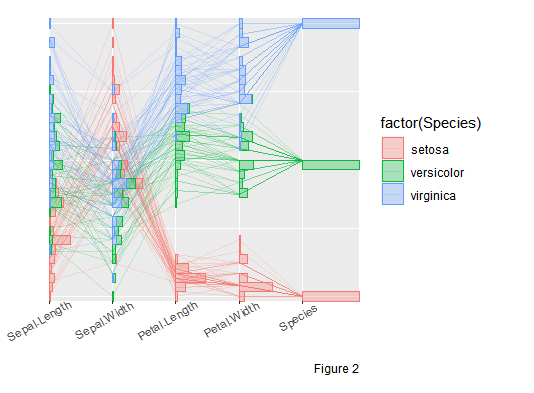A density layer can be drawn by adding layer geom_density

p +
geom_density(alpha = 0.3,
mapping = aes(fill = factor(Species)))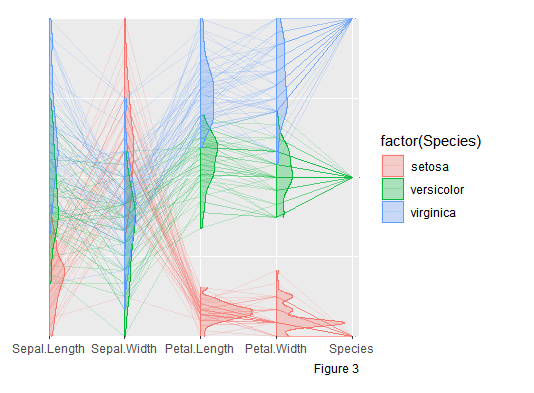A parallel coordinate can be converted to radial coordinate by setting axes.layout = "radial" in function coord_serialaxes.

p$coordinates$axes.layout <- "radial"
p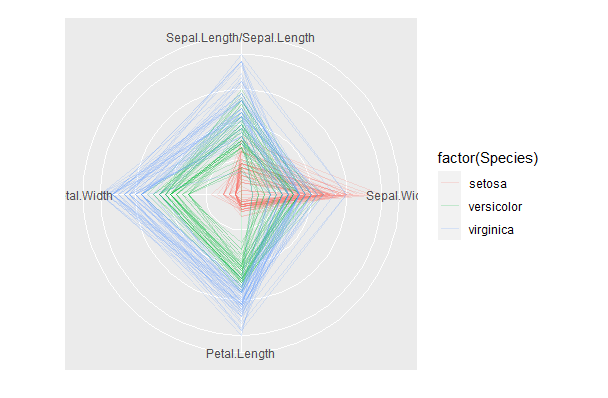Note that: layers, such as geom_histogram, geom_density, etc, are not implemented in the radial coordinate yet.

### Infinite axes

Andrews (1972) plot is a way to project multi-response observations into a function $$f(t)$$, by defining $$f(t)$$ as an inner product of the observed values of responses and orthonormal functions in $$t$$

$f_{y_i}(t) = <\mathbf{y}_i, \mathbf{a}_t>$

where $$\mathbf{y}_i$$ is the $$i$$th responses and $$\mathbf{a}_t$$ is the orthonormal functions under certain interval. Andrew suggests to use the Fourier transformation

$\mathbf{a}_t = \{\frac{1}{\sqrt{2}}, \sin(t), \cos(t), \sin(2t), \cos(2t), ...\}$

which are orthonormal on interval $$(-\pi, \pi)$$. In other word, we can project a $$p$$ dimensional space to an infinite $$(-\pi, \pi)$$ space. The following figure illustrates how to construct an “Andrew’s plot”.

p <- ggplot(iris,
mapping = aes(Sepal.Length = Sepal.Length,
Sepal.Width = Sepal.Width,
Petal.Length = Petal.Length,
Petal.Width = Petal.Width,
colour = Species)) +
geom_path(alpha = 0.2,
stat = "dotProduct",
# no scaling is applied
scaling = "none")  +
coord_serialaxes()
p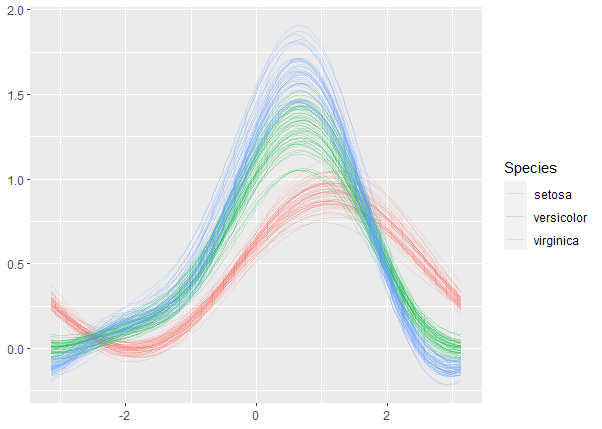A quantile layer can be displayed on top

p +
geom_quantiles(stat = "dotProduct",
scaling = "none",
quantiles = c(0.25, 0.5, 0.75),
size = 2,
linetype = 2)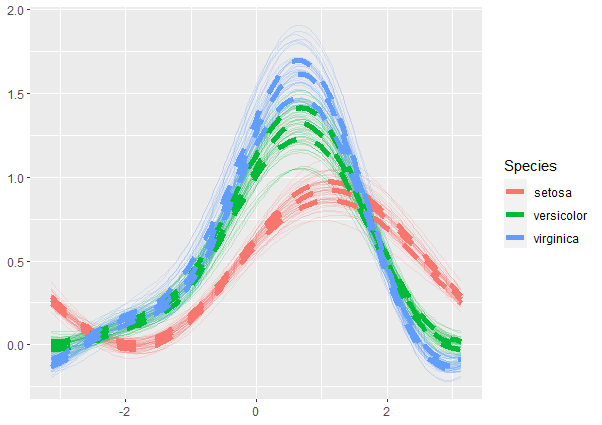A couple of things should be noticed:

• mapping aesthetics is used to define the $$p$$ dimensional space, if not provided, all columns in the dataset ‘iris’ will be transformed. An alternative way to determine the $$p$$ dimensional space to set parameter axes.sequence in each layer or in coord_serialaxes.

• To construct a dot product serial axes plot, say Fourier transformation, “Andrew’s plot”, we need to set the parameter stat in geom_path to “dotProduct”. The default transformation function is the Andrew’s (function andrews). Users can customize their own, for example, Tukey suggests the following projected space

$\mathbf{a}_t = \{\cos(t), \cos(\sqrt{2}t), \cos(\sqrt{3}t), \cos(\sqrt{5}t), ...\}$

where $$t \in [0, k\pi]$$ (Gnanadesikan 2011).

tukey <- function(p = 4, k = 50 * (p - 1), ...) {
t <- seq(0, p* base::pi, length.out = k)
seq_k <- seq(p)
values <- sapply(seq_k,
function(i) {
if(i == 1) return(cos(t))
if(i == 2) return(cos(sqrt(2) * t))
Fibonacci <- seq_k[i - 1] + seq_k[i - 2]
cos(sqrt(Fibonacci) * t)
})
list(
vector = t,
matrix = matrix(values, nrow = p, byrow = TRUE)
)
}
ggplot(iris,
mapping = aes(Sepal.Length = Sepal.Length,
Sepal.Width = Sepal.Width,
Petal.Length = Petal.Length,
Petal.Width = Petal.Width,
colour = Species)) +
geom_path(alpha = 0.2, stat = "dotProduct", transform = tukey)  +
coord_serialaxes()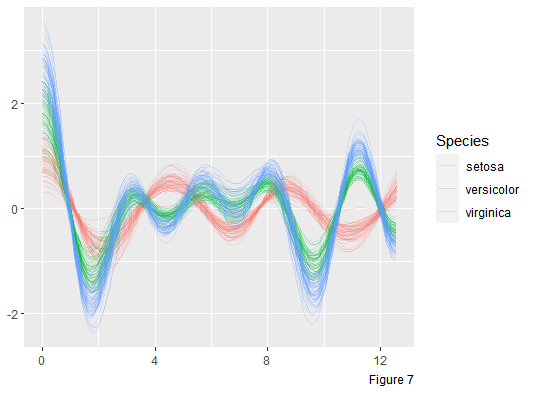Note that: Tukey’s suggestion, element $$\mathbf{a}_t$$ can “cover” more spheres in $$p$$ dimensional space, but it is not orthonormal.

### An alternative way to create a serial axes plot

Rather than calling function coord_serialaxes, an alternative way to create a serial axes object is to add a geom_serialaxes_... object in our model.

For example, Figure 1 to 4 can be created by calling

g <- ggplot(iris,
mapping = aes(Sepal.Length = Sepal.Length,
Sepal.Width = Sepal.Width,
Petal.Length = Petal.Length,
Petal.Width = Petal.Width,
colour = Species))
g + geom_serialaxes(alpha = 0.2)
g +
geom_serialaxes(alpha = 0.2) +
geom_serialaxes_hist(mapping = aes(fill = Species), alpha = 0.2)
g +
geom_serialaxes(alpha = 0.2) +
geom_serialaxes_density(mapping = aes(fill = Species), alpha = 0.2)
# radial axes can be created by
# calling coord_radar() or coord_polar(is_linear = TRUE)
# this is slightly different, check it out!
g +
geom_serialaxes(alpha = 0.2) +
geom_serialaxes(alpha = 0.2) +
coord_radar()

Figure 5 and 7 can be created by setting “stat” and “transform” in geom_serialaxes; to Figure 6, geom_serialaxes_quantile can be added to create a serial axes quantile layer.

Some slight difference should be noticed here:

• One benefit of calling coord_serialaxes rather than geom_serialaxes_... is that coord_serialaxes can accommodate duplicated axes in mapping aesthetics (e.g. Eulerian path, Hamiltonian path, etc). However, in geom_serialaxes_..., duplicated axes will be omitted.

• Meaningful axes labels in coord_serialaxes can be created automatically, while in geom_serialaxes_..., users have to set axes labels by ggplot2::scale_x_continuous or ggplot2::scale_y_continuous manually.

• As we turn the serial axes into interactive graphics (via package loon.ggplot), serial axes lines in coord_serialaxes() could be turned as interactive but in geom_serialaxes_... all objects are static.

# The serial axes is Sepal.Length, Sepal.Width, Sepal.Length
# With meaningful labels
ggplot(iris,
mapping = aes(Sepal.Length = Sepal.Length,
Sepal.Width = Sepal.Width,
Sepal.Length = Sepal.Length)) +
geom_path() +
coord_serialaxes()

# The serial axes is Sepal.Length, Sepal.Length
# No meaningful labels
ggplot(iris,
mapping = aes(Sepal.Length = Sepal.Length,
Sepal.Width = Sepal.Width,
Sepal.Length = Sepal.Length)) +
geom_serialaxes()

Also, if the dimension of data is large, typing each variate in mapping aesthetics is such a headache. Parameter axes.sequence is provided to determine the axes. For example, a serialaxes object can be created as

ggplot(iris) +
geom_path() +
coord_serialaxes(axes.sequence = colnames(iris)[-5])

At very end, please report bugs here. Enjoy the high dimensional visualization! “Don’t panic… Just do it in ‘serial’” (Inselberg 1999).

## Reference

Andrews, David F. 1972. “Plots of High-Dimensional Data.” Biometrics, 125–0136.

Gnanadesikan, Ram. 2011. “Methods for Statistical Data Analysis of Multivariate Observations.” In, 321:207–0218. John Wiley & Sons.

Inselberg, A., and B. Dimsdale. 1990. “Parallel Coordinates: A Tool for Visualizing Multi-Dimensional Geometry.” In Proceedings of the First Ieee Conference on Visualization: Visualization ‘90, 361–0378.

Inselberg, Alfred. 1999. “Don’t Panic... Just Do It in Parallel!” Computational Statistics 14 (1): 53–077.